## Overview

Movement is a very basic concept in video games, it could be as simple as accumulating steps to a position value. This article introduces a smooth movement algorithm with tolerant sliding, which offers good adaptivity to various tile-based scenes.

## Objective

Remember how smooth the control was when playing the Bomberman?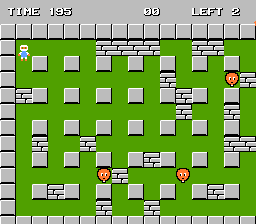Bomberman

If press right, for example, while there is a block tile near your bomberman's feet, the character will try moving into his offset direction (relative to the block) on another axis to avoid blocking, then continue into the desired direction.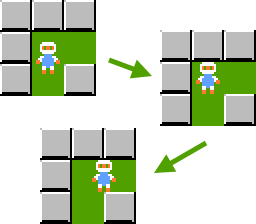Pressing right

Our goal is to reproduce this tactile feel and furthermore make it adaptable to different character and tile size.

## Grid-stepped movement

Starting from the simplest attempt, assuming we got a small tile map: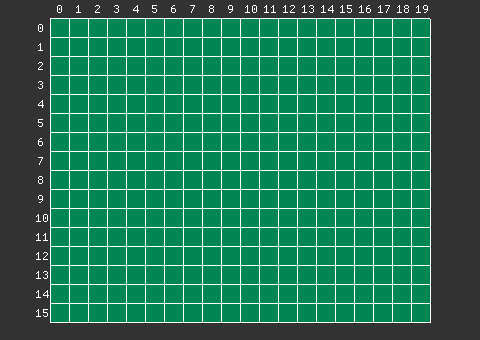Tile map

Position could be abstracted to dual integer values for x, y respectively. Then the moving and rendering code would be:

``````local pos = Vec2.new(0, 0)
function update()
-- Grid-wise move.
if keyp(KeyCode.Left) then
pos.x = pos.x - 1
elseif keyp(KeyCode.Right) then
pos.x = pos.x + 1
end
if keyp(KeyCode.Up) then
pos.y = pos.y - 1
elseif keyp(KeyCode.Down) then
pos.y = pos.y + 1
end
-- Pixelated rendering.
map(ground, 0, 0)
spr(hero, pos.x * TILE_SIZE, pos.y * TILE_SIZE)
end``````

Object position and tile index share same grid unit in this situation.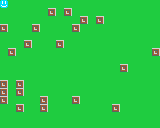Grid-stepped

## Pixel-stepped movement

The grid-stepped code can be modified to a pixel-stepped version by simply changing the last sprite rendering line:

``````local pos = Vec2.new(0, 0)
function update()
-- Pixelated move.
if key(KeyCode.Left) then
pos.x = pos.x - 1
elseif key(KeyCode.Right) then
pos.x = pos.x + 1
end
if key(KeyCode.Up) then
pos.y = pos.y - 1
elseif key(KeyCode.Down) then
pos.y = pos.y + 1
end
-- Pixelated rendering.
map(ground, 0, 0)
spr(hero, pos.x, pos.y)
end``````

Now object position uses pixel unit same to rendering. It looks smoother than the previous version.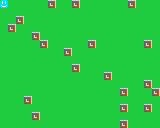Pixel-stepped

## Collision detection

We didn't count any time factor in this ideal pseudo code, but the later code looks even simpler than the former, how come? Consider adding collision detection against tiles to tell whether it's movable. The code shall be:

``````-- Grid-stepped.
function isMovable()
local index = pos + expDir
local tile = mget(ground, index.x, index.y)
local movable = not isBlock(tile)
return movable
end``````

We need to apply a pixel-to-grid conversion before accessing map tiles for pixel-stepped:

``````-- Pixel-stepped.
function isMovable()
local newPos = pos + expDir
local index = Vec2.new(
math.floor(newPos.x / TILE_SIZE),
math.floor(newPos.y / TILE_SIZE)
)
local tile = mget(ground, index.x, index.y)
local movable = not isBlock(tile)
return movable
end``````

Things are getting more complicated from here, but no need to worry.

The position is yet no longer truncated in grids, we can't determine whether it's movable or not unless checking all the possible swept ways ahead a character's front edge of movement.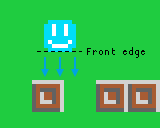Multiple scan lines

We may as well call it "scan line" in this article. In real projects, more scan lines means more CPU consumption. The good news is that we can simplify this evaluation to several checkings with gap rather than doing that for every pixel line.

``````local STEP_COUNT = 3
function isVerticallyMovable()
local step = CHAR_SIZE / STEP_COUNT
local beginX = pos.x + expDir.x
local endX = pos.x + expDir.x + CHAR_SIZE
local movable = true
for x = beginX, endX, step do
local newPos = pos + Vec2.new(x, pos.y + expDir.y)
local index = Vec2.new(
math.floor(newPos.x / TILE_SIZE),
math.floor(newPos.y / TILE_SIZE)
)
local tile = mget(ground, index.x, index.y)
if isBlock(tile) then
movable = false
break
end
end
return movable
end``````

The additional benefit is that this algorithm also works with different character and tile size now.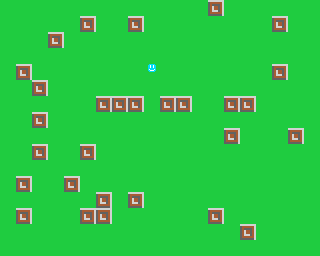Small character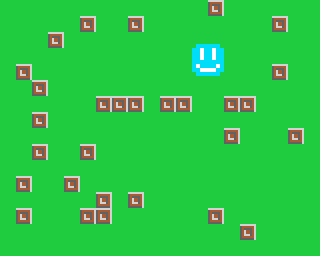Big character

## Sliding optimization

You still have to align your character carefully to walk through a narrow passage until now. To optimize the behaviour, first shrink the bundle width of scan lines to fit for tolerance.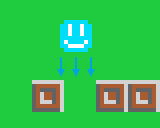Shrink for tolerance

Secondly, calculate a movable rate to tell how much it's passable through.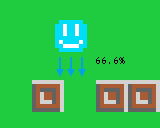Movable rate

Passed count of scan lines divides total count for the rate:

``````local SHRINK_SIZE = 1
local MOVABLE_RATE = 0.6667
local STEP_COUNT = 3
function isVerticallyMovable()
local step = (CHAR_SIZE - SHRINK_SIZE * 2) / STEP_COUNT
local beginX = pos.x + expDir.x + SHRINK_SIZE
local endX = pos.x + expDir.x + CHAR_SIZE - SHRINK_SIZE
local passed = 0
local total = 0
for x = beginX, endX, step do
local newPos = pos + Vec2.new(x, pos.y + expDir.y)
local index = Vec2.new(
math.floor(newPos.x / TILE_SIZE),
math.floor(newPos.y / TILE_SIZE)
)
local tile = mget(ground, index.x, index.y)
if not isBlock(tile) then
passed = passed + 1
end
total = total + 1
end
local movable = passed / total >= MOVABLE_RATE
return movable
end``````

And eventually, if the rate is sufficient to move but still blocked by something, we do a sliding on the other axis to make a minor correction for further movement.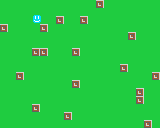Smooth movement

## One-way platform

In some games, you can jump through upward a platform, then the platform supports you from falling down; or you walk through a door but there's no way back... We call this a one-way platform or one-way door. We can achieve this behaviour by adding a mask parameter for conditional block.

``````local NONE, LEFT, RIGHT, UP, DOWN = 0, 1, 2, 4, 8
...
...
for x = beginX, endX, step do
...
local mask = expDir.y < 0 and UP or DOWN
passed = passed + 1
end
total = total + 1
end
local movable = passed / total >= MOVABLE_RATE
return movable
end``````

Now it becomes upward movable when pass `UP` to the function.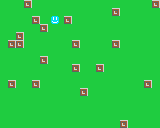One-way platform

## Applications

This algorithm works smoothly in top-down and platformer games. And the idea also fits well in properly organized 3D scenes.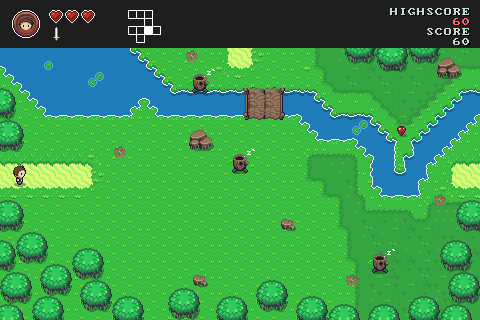Top-down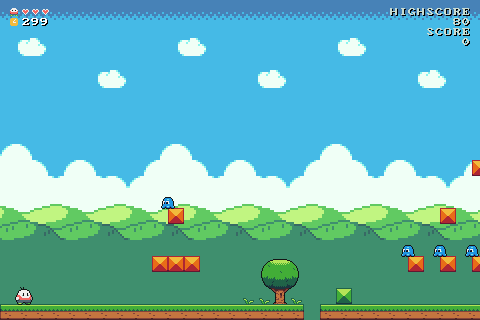Platformer

## Known issues

### Penetration problem

This algorithm doesn't forbid penetration through a tile if a single step is greater than the tile size. However, you can split a big step into several sub steps to avoid undesired penetration.

### Real number precision

The precision of IEEE-754 standardized real numbers are limited. An issue will manifest when move at the border area of a big map. It is inaccurate to add a small step number to a big position number. Using double precision instead of single precision would relief it, but it only respites the inaccuracy till a larger scale. A workable solution is to apply offset to everything (including characters, map, camera, etc.) to project values inside a computable range all the way.

## Gist Code

You are free to copy, use and port this `Walker` algorithm code in C++ under the BSD 3-Clause License. Replace the `Math` and `Vec2` polyfill however you want in your actual project.

Implementation:

``````/*
**
**
** Redistribution and use in source and binary forms, with or without
** modification, are permitted provided that the following conditions are met:
**
** * Redistributions of source code must retain the above copyright notice, this
**   list of conditions and the following disclaimer.
**
** * Redistributions in binary form must reproduce the above copyright notice,
**   this list of conditions and the following disclaimer in the documentation
**   and/or other materials provided with the distribution.
**
** * Neither the name of the copyright holder nor the names of its
**   contributors may be used to endorse or promote products derived from
**   this software without specific prior written permission.
**
** THIS SOFTWARE IS PROVIDED BY THE COPYRIGHT HOLDERS AND CONTRIBUTORS "AS IS"
** AND ANY EXPRESS OR IMPLIED WARRANTIES, INCLUDING, BUT NOT LIMITED TO, THE
** IMPLIED WARRANTIES OF MERCHANTABILITY AND FITNESS FOR A PARTICULAR PURPOSE ARE
** DISCLAIMED. IN NO EVENT SHALL THE COPYRIGHT HOLDER OR CONTRIBUTORS BE LIABLE
** FOR ANY DIRECT, INDIRECT, INCIDENTAL, SPECIAL, EXEMPLARY, OR CONSEQUENTIAL
** DAMAGES (INCLUDING, BUT NOT LIMITED TO, PROCUREMENT OF SUBSTITUTE GOODS OR
** SERVICES; LOSS OF USE, DATA, OR PROFITS; OR BUSINESS INTERRUPTION) HOWEVER
** CAUSED AND ON ANY THEORY OF LIABILITY, WHETHER IN CONTRACT, STRICT LIABILITY,
** OR TORT (INCLUDING NEGLIGENCE OR OTHERWISE) ARISING IN ANY WAY OUT OF THE USE
** OF THIS SOFTWARE, EVEN IF ADVISED OF THE POSSIBILITY OF SUCH DAMAGE.
*/
#ifndef __WALKER_H__
#define __WALKER_H__
#include <cmath>
#include <functional>
#ifndef GRID_DEFAULT_SIZE
#  define GRID_DEFAULT_SIZE 8
#endif /* GRID_DEFAULT_SIZE */
struct Math {
template<typename T> static int sign(T v) {
if (v < 0)
return -1;
else if (v > 0)
return 1;
return 0;
}
};
typedef float Real;
template<typename T, typename R = Real> struct Vec2 {
T x = 0;
T y = 0;
Vec2() {
}
Vec2(T x_, T y_) : x(x_), y(y_) {
}
Vec2(const Vec2 &other) {
x = other.x;
y = other.y;
}
Vec2 &operator = (const Vec2 &other) {
x = other.x;
y = other.y;
return *this;
}
bool operator == (const Vec2 &other) const {
return x == other.x && y == other.y;
}
bool operator != (const Vec2 &other) const {
return x != other.x || y != other.y;
}
R length(void) const {
return std::sqrt((R)(x * x + y * y));
}
};
typedef Vec2<Real> Vec2f;
typedef Vec2<int> Vec2i;
class Walker {
public:
enum Directions {
NONE = 0,
LEFT = 1 << 0,
RIGHT = 1 << 1,
UP = 1 << 2,
DOWN = 1 << 3
};
struct Blocking {
bool block = false;
unsigned pass = 0;
Blocking() {
}
Blocking(bool blk, unsigned pass_) : block(blk), pass(pass_) {
}
};
typedef std::function<Blocking(const Vec2i &)> BlockingHandler;
public:
Walker() {
}
~Walker() {
}
Vec2i objectSize(void) const {
return _objSize;
}
void objectSize(const Vec2i &size) {
_objSize = size;
}
Vec2i tileSize(void) const {
return _tileSize;
}
void tileSize(const Vec2i &size) {
_tileSize = size;
}
Vec2f offset(void) const {
return _offset;
}
void offset(const Vec2f &offset) {
_offset = offset;
}
/**
* @brief Solves whether a specific object is movable into a direction, and
*   tells the movable vector.
*
* @param[in] objPos The object position.
* @param[in] expDir The expected direction vector.
* @param[in] block A function which evaluates walkable state in scene.
* @param[out] newDir Evaluated movement vector.
* @param[in] slidable The slidable factor.
* @return Positive for movement length, or 0 for blocked.
*/
int solve(
const Vec2f &objPos, const Vec2f &expDir,
BlockingHandler block,
Vec2f &newDir,
int slidable = 5
) {
if (_objSize == Vec2i(0, 0))
return 0;
if (_tileSize == Vec2i(0, 0))
return 0;
const Real expDirX = expDir.x, expDirY = expDir.y;
int n = tend( // Tend straightforward.
objPos, expDir,
block,
newDir,
slidable,
&_objSize, &_tileSize, &_offset
);
if (!n)
return n;
if (!slidable)
return n;
if (Math::sign(expDirX) != Math::sign(newDir.x) || Math::sign(expDirY) != Math::sign(newDir.y)) { // The movement has been redirected.
const Vec2f newExpDir(newDir.x, newDir.y);
Vec2f newNewDir(0, 0);
n = tend( // Tend into a new direction.
objPos, newExpDir,
block,
newNewDir,
slidable,
&_objSize, &_tileSize, &_offset
);
if (!n)
return n;
if (Math::sign(newDir.x) != Math::sign(newNewDir.x) || Math::sign(newDir.y) != Math::sign(newNewDir.y)) // Neither passable.
return 0;
}
return n;
}
private:
template<typename T = Real> static int tend(
const Vec2f &objPos, const Vec2f &expDir,
BlockingHandler block,
Vec2f &newDir,
int slidable,
const Vec2i* objSize_, const Vec2i* tileSize_, const Vec2f* offset
) {
// Prepare.
typedef T Number;
constexpr const Number EPSILON = Number(0.000001f);
constexpr const Number MARGIN = Number(1.001f);
const Vec2i objSize = objSize_ ? *objSize_ : Vec2i(GRID_DEFAULT_SIZE, GRID_DEFAULT_SIZE);
const Vec2i tileSize = tileSize_ ? *tileSize_ : Vec2i(GRID_DEFAULT_SIZE, GRID_DEFAULT_SIZE);
if (expDir.x == 0 && expDir.y == 0) {
newDir.x = newDir.y = 0;
return 0;
}
// Calculate the edges and center position.
const Number objWidth = Number(objSize.x);
const Number objHeight = Number(objSize.y);
const Number objLocalX0 = -objWidth / 2;
const Number objLocalX1 = objWidth / 2;
const Number objLocalY0 = -objHeight / 2;
const Number objLocalY1 = objHeight / 2;
Number centerX = Number(objPos.x) + objWidth / 2 + Number(expDir.x);
Number centerY = Number(objPos.y) + objHeight / 2 + Number(expDir.y);
if (offset) {
centerX -= Number(offset->x);
centerY -= Number(offset->y);
}
// Resolve.
Number dirX = Number(expDir.x);
Number dirY = Number(expDir.y);
Number dampingX = Number(0);
Number dampingY = Number(0);
const Number stepHeight = Number(objHeight - MARGIN * 2);
const Number stepY = Number(stepHeight / std::ceil(stepHeight / tileSize.y));
if (dirX > Number(0)) {
int total = 0;
int blocked = 0;
Number diffX = 0;
const Number frontX = centerX + objLocalX1;
for (Number j = Number(objLocalY0 + MARGIN); j < objLocalY1; j += stepY) {
const Number frontY = centerY + j;
const int frontTileIdxX = (int)std::floor(frontX / tileSize.x);
const int frontTileIdxY = (int)std::floor(frontY / tileSize.y);
const Blocking blk = block(Vec2i(frontTileIdxX, frontTileIdxY));
if (blk.block && !(blk.pass & RIGHT)) {
const Number diff = frontTileIdxX * tileSize.x - frontX;
if (diff < diffX)
diffX = diff;
dampingX -= Math::sign(j) * std::abs(diff);
++blocked;
}
++total;
}
if (diffX < Number(0)) {
dirX += diffX;
if (std::abs(dirX) <= EPSILON)
dirX = Number(0);
}
if (dirX < Number(0))
dirX = Number(0);
if (Number(blocked) / total * 10 > slidable)
dampingX = 0;
} else if (dirX < Number(0)) {
int total = 0;
int blocked = 0;
Number diffX = 0;
const Number frontX = centerX + objLocalX0;
for (Number j = Number(objLocalY0 + MARGIN); j < objLocalY1; j += stepY) {
const Number frontY = centerY + j;
const int frontTileIdxX = (int)std::floor(frontX / tileSize.x);
const int frontTileIdxY = (int)std::floor(frontY / tileSize.y);
const Blocking blk = block(Vec2i(frontTileIdxX, frontTileIdxY));
if (blk.block && !(blk.pass & LEFT)) {
const Number diff = frontTileIdxX * tileSize.x + tileSize.x - frontX;
if (diff > diffX)
diffX = diff;
dampingX -= Math::sign(j) * std::abs(diff);
++blocked;
}
++total;
}
if (diffX > Number(0)) {
dirX += diffX;
if (std::abs(dirX) <= EPSILON)
dirX = Number(0);
}
if (dirX > Number(0))
dirX = Number(0);
if (Number(blocked) / total * 10 > slidable)
dampingX = 0;
}
const Number stepWidth = Number(objWidth - MARGIN * 2);
const Number stepX = Number(stepWidth / std::ceil(stepWidth / tileSize.x));
if (dirY > Number(0)) {
int total = 0;
int blocked = 0;
Number diffY = 0;
const Number frontY = centerY + objLocalY1;
for (Number i = Number(objLocalX0 + MARGIN); i < objLocalX1; i += stepX) {
const Number frontX = centerX + i;
const int frontTileIdxX = (int)std::floor(frontX / tileSize.x);
const int frontTileIdxY = (int)std::floor(frontY / tileSize.y);
const Blocking blk = block(Vec2i(frontTileIdxX, frontTileIdxY));
if (blk.block && !(blk.pass & DOWN)) {
const Number diff = frontTileIdxY * tileSize.y - frontY;
if (diff < diffY)
diffY = diff;
dampingY -= Math::sign(i) * std::abs(diff);
++blocked;
}
++total;
}
if (diffY < Number(0)) {
dirY += diffY;
if (std::abs(dirY) <= EPSILON)
dirY = Number(0);
}
if (dirY < Number(0))
dirY = Number(0);
if (Number(blocked) / total * 10 > slidable)
dampingY = 0;
} else if (dirY < Number(0)) {
int total = 0;
int blocked = 0;
Number diffY = 0;
const Number frontY = centerY + objLocalY0;
for (Number i = Number(objLocalX0 + MARGIN); i < objLocalX1; i += stepX) {
const Number frontX = centerX + i;
const int frontTileIdxX = (int)std::floor(frontX / tileSize.x);
const int frontTileIdxY = (int)std::floor(frontY / tileSize.y);
const Blocking blk = block(Vec2i(frontTileIdxX, frontTileIdxY));
if (blk.block && !(blk.pass & UP)) {
const Number diff = frontTileIdxY * tileSize.y + tileSize.y - frontY;
if (diff > diffY)
diffY = diff;
dampingY -= Math::sign(i) * std::abs(diff);
++blocked;
}
++total;
}
if (diffY > Number(0)) {
dirY += diffY;
if (std::abs(dirY) <= EPSILON)
dirY = Number(0);
}
if (dirY > Number(0))
dirY = Number(0);
if (Number(blocked) / total * 10 > slidable)
dampingY = 0;
}
// Slide.
if (slidable) {
if (dirX == Number(0) && expDir.x != 0 && expDir.y == 0) {
if (dampingX == Number(0)) {
dirY = Number(0);
} else {
const Number expDirX = Number(expDir.x);
Number frontX = Number(0);
if (expDirX > Number(0))
frontX = centerX + objLocalX1;
else if (expDirX < Number(0))
frontX = centerX + objLocalX0;
if (expDirX != Number(0)) {
const Number frontY = centerY;
const int frontTileIdxX = (int)std::floor(frontX / tileSize.x);
const int frontTileIdxY = (int)std::floor(frontY / tileSize.y);
const Blocking blk = block(Vec2i(frontTileIdxX, frontTileIdxY));
if (!blk.block) {
if (dampingX < Number(0))
dirY = (frontTileIdxY + 1) * tileSize.y - (frontY + objLocalY1);
else /* if (dampingX > Number(0)) */
dirY = frontTileIdxY * tileSize.y - (frontY + objLocalY0);
if (std::abs(dirY) > std::abs(expDirX))
dirY = Math::sign(dirY) * std::abs(expDirX);
}
}
}
}
if (dirY == Number(0) && expDir.y != 0 && expDir.x == 0) {
if (dampingY == Number(0)) {
dirX = Number(0);
} else {
const Number expDirY = Number(expDir.y);
Number frontY = Number(0);
if (expDirY > Number(0))
frontY = centerY + objLocalY1;
else if (expDirY < Number(0))
frontY = centerY + objLocalY0;
if (expDirY != Number(0)) {
const Number frontX = centerX;
const int frontTileIdxX = (int)std::floor(frontX / tileSize.x);
const int frontTileIdxY = (int)std::floor(frontY / tileSize.y);
const Blocking blk = block(Vec2i(frontTileIdxX, frontTileIdxY));
if (!blk.block) {
if (dampingY < Number(0))
dirX = (frontTileIdxX + 1) * tileSize.x - (frontX + objLocalX1);
else /* if (dampingY > Number(0)) */
dirX = frontTileIdxX * tileSize.x - (frontX + objLocalX0);
if (std::abs(dirX) > std::abs(expDirY))
dirX = Math::sign(dirX) * std::abs(expDirY);
}
}
}
}
}
// Accept.
newDir = Vec2f((Real)dirX, (Real)dirY);
return (int)newDir.length();
}
private:
Vec2i _objSize = Vec2i(GRID_DEFAULT_SIZE, GRID_DEFAULT_SIZE);
Vec2i _tileSize = Vec2i(GRID_DEFAULT_SIZE, GRID_DEFAULT_SIZE);
Vec2f _offset = Vec2f(0, 0);
};
#endif /* __WALKER_H__ */``````

Usage:

``````Walker* walker = new Walker();
const Vec2f objPos(...);
const Vec2f expDir(...);
Vec2f newDir;
walker->solve(
objPos, expDir,
[] (const Vec2i &pos) -> Walker::Blocking {
return Walker::Blocking(false, 0);
},
newDir,
5
);
delete walker;``````